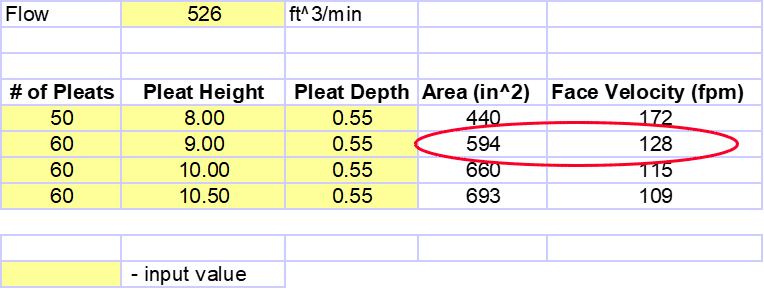Go Back To Home

# Air Filter Selection

It is important to appropriately size the air filter for the maximum flow rate of the application. A target face velocity of ≤130 ft/min at redline is used to minimize restriction and to provide the turbo with the air necessary for it to function optimally. If the turbo does not have access to the proper amount of air, excessive restriction will occur and cause:

• Oil leakage from the compressor side piston ring, which results in oil loss, a fouled intercooler and potentially smoke out of the tailpipe.
• Increased pressure ratio, which can lead to turbo overspeed.
• Overspeed will reduce turbo durability and could result in early turbo failure.

### Determining the correct air filter size

Example:
Face Velocity = 130 ft/min
Mass Flow = 40 lbs/min
Air density = 0.076 lbs/ft³
Mass Flow (lbs/min) = Volumetric Flow Rate (CFM) x Air Density (lbs/ft³)

Volumetric Flow Rate (CFM) = Mass Flow (lbs/mn) / Air Density (lbs/ft³)

Volumetric Flow Rate = 526 CFM
For twin applications divide the flow rate by 2

Face Velocity (ft/min) = Volumetric Flow Rate (CFM) / Area (ft²)

Area (ft²) = CFM / Face Velocity (ft/min)

Area (ft²) = 526 / 130 = 4.05

Area (in²) = 4.05 x 144

Area= 582 in²

How to determine filter size once you know the calculated area

Area (in²) = pleat height x pleat depth x number of pleats x 2

Area (in²) = 9.00 x .55 x 60 x 2

Area = 594 in²

Actual Filter Area (594 in²) > Calculated Area (582 in²)